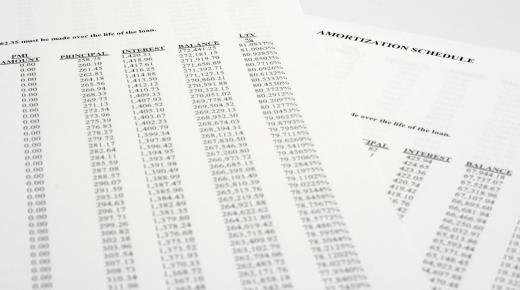Finance
Fact Checked

# How does an Amortization Formula Work?

Danielle DeLee
Danielle DeLee

An amortization formula is based on the formula for calculating the value of an annuity. From this basic formula, you can determine the monthly payment on a fully amortizing loan. You can further modify it to get formulas that yield the remaining principal, the principal paid in a particular month, the interest paid in a particular month and the total interest on the loan.

The formula for determining the present value of an annuity is PV = C/r*(1-1/(1+r)T). PV is the present value, C is the coupon, r is the interest rate and T is the number of periods in the life of the loan. One consideration is that the interest rate must be adjusted to suit the period length. Usually, the rate is given in an annuity contract as an annualized figure. To get the interest rate for one period, divide the annualized rate by the number of payment periods in one year.With an amortization formula, you can determine the monthly payment on a fully amortizing loan.

This formula is also used as an amortization formula because amortizing loans function in the same way that annuities do. When you purchase an annuity, you trade a lump sum for the promise of a stream of equally sized payments at regular periods. In an amortizing loan, the lender gives a lump sum to the borrower in exchange for a series of monthly payments, in effect purchasing an annuity from the borrower.

To determine the monthly payment on an amortizing loan, like a mortgage, just use the numbers for the loan in the original amortization formula. If a borrower gets a \$300,000 US Dollars (USD) mortgage for 30 years at 6 percent, for example, you would plug 300,000 in for PV. The interest rate would be the mortgage rate, 6 percent, divided by 12, which equals 0.5 percent. T would be 30 years times 12 payments per year, which is 360. Then, you can solve the equation for C, which is the monthly payment on the loan.

The remaining balance before each payment is made multiplied by the periodic interest rate gives the amount of the monthly payment that goes toward interest. The remainder of the payment goes toward principal. You can use the basic amortization formula to construct an amortization schedule, which shows the amount of principal that is paid off in each monthly payment. The formula can also be used to derive formulas that allow you to calculate the information contained in an amortization schedule for any given period without writing out the full schedule.

## You might also Like